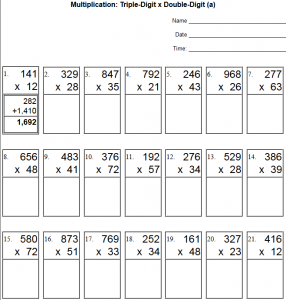# Multi-digit Multiplication WorksheetsPrintable Multi-Digit Multiplication Worksheets - Free printable algorithm double-digit times single-digit, double-digit times double-digit, triple-digit times single-digit, triple-digit times double-digit, and triple-digit times triple-digit multiplication worksheets.

CCSS.Math.Content.4.NBT.B.5
Multiply a whole number of up to four digits by a one-digit whole number, and multiply two two-digit numbers, using strategies based on place value and the properties of operations. Illustrate and explain the calculation by using equations, rectangular arrays, and/or area models.
CCSS.Math.Content.5.NBT.B.5 Fluently multiply multi-digit whole numbers using the standard algorithm.